# x - y + 2z + w = +4 -2x + 3y = -4 x +...

x - y + 2z + w = +4

-2x + 3y = -4

x + y + z - 4w = +3

Rewrite linear system as matrix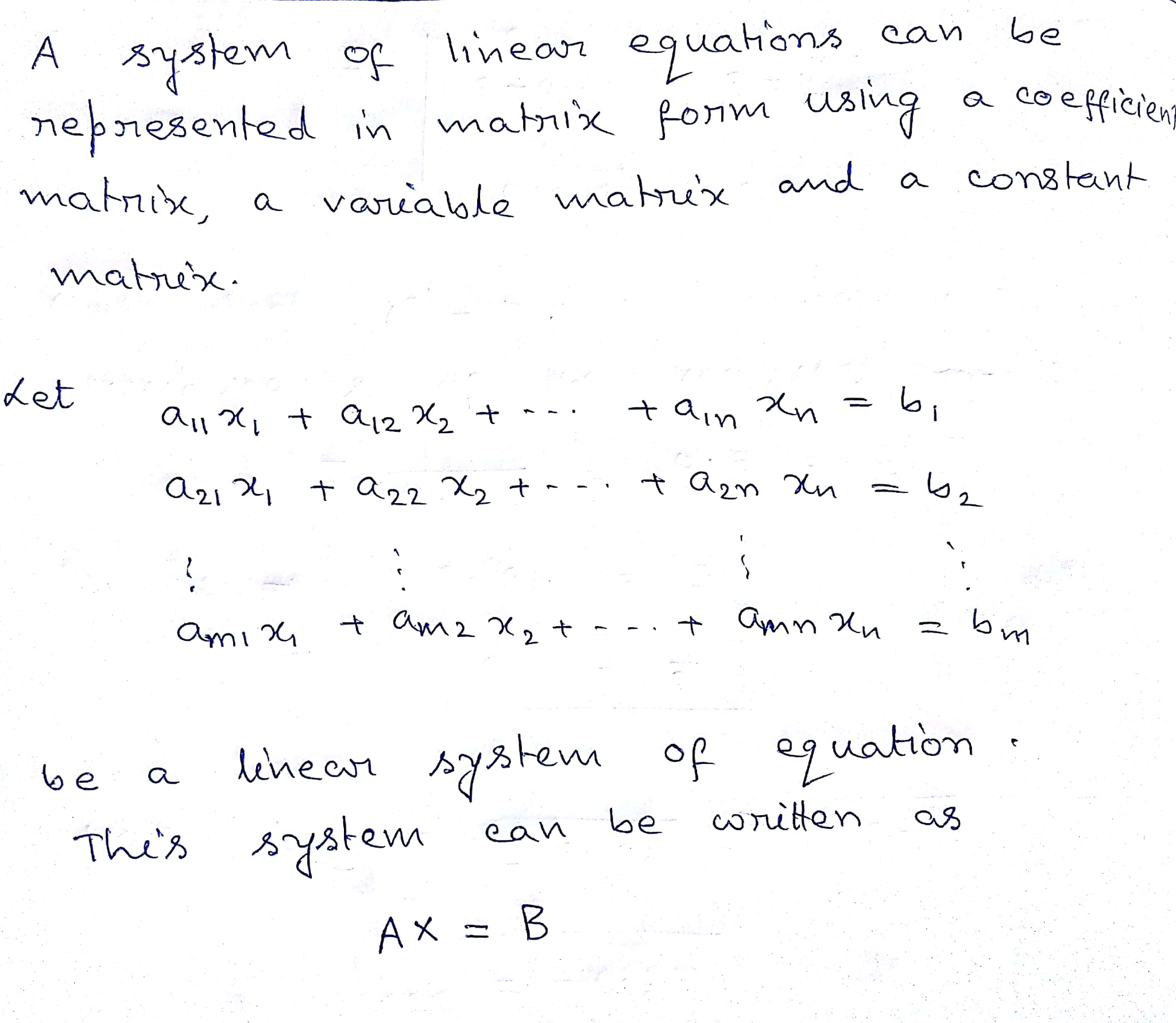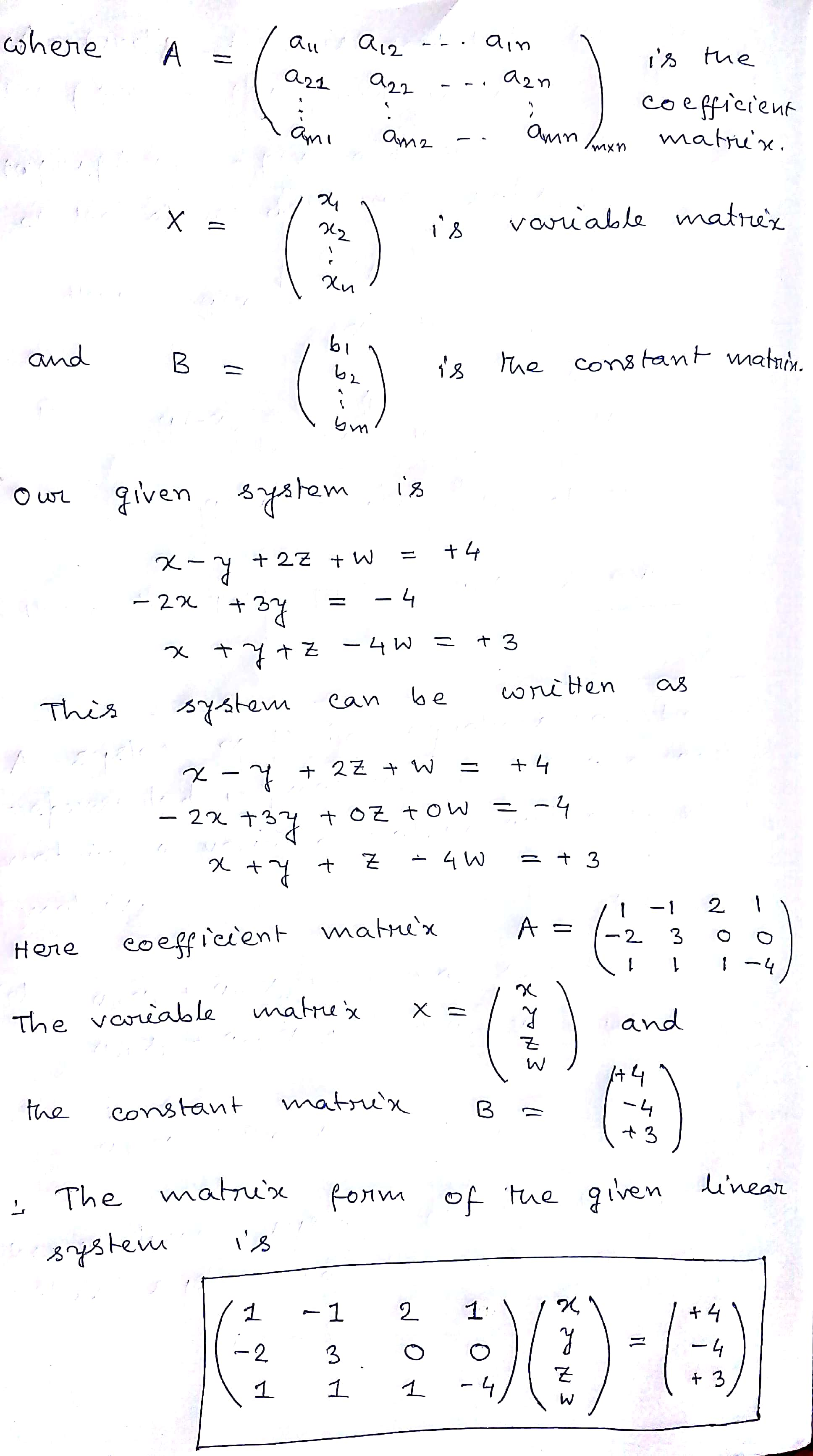##### Add Answer of: x - y + 2z + w = +4 -2x + 3y = -4 x +...
Similar Homework Help Questions
• ### determine if the following set of equations has infinitely many solutions or no solutions x-y+2z+w =...

determine if the following set of equations has infinitely many solutions or no solutions x-y+2z+w = 4 -2x+3y=-4 x+y+z-4w=3

• ### -x+y+3z=6 x+y+2z=7 2x+3y+z=4

-x+y+3z=6 x+y+2z=7 2x+3y+z=4

• ### solve the system of equations for all variables using a matrix x-y-z=1 2x+3y+z=10 x+y-2z=0 can not have fraction

solve the system of equations for all variables using a matrixx-y-z=12x+3y+z=10x+y-2z=0can not have fraction

• ### Consider the linear system x + 2y ? z = ?7 2x ? 2y ? 2z = ?8 ?x + 3y + 4z = 8 and solve it by...

Consider the linear systemx + 2y - z = -72x - 2y - 2z = -8-x + 3y + 4z = 8and solve it by Cramer’s Rule.Let A be the determinant of the coef?cient matrix. ThenA = .Moreover,x =A1A where A1 = .y =A2A where A2 = .z =A3A where A3 = .

• ### linear algebra Determine the augmented matrix A# of the given system. W + 2 2x +...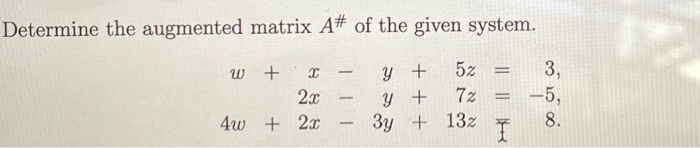linear algebra Determine the augmented matrix A# of the given system. W + 2 2x + 2x – – - y y 3y + + + 5z = 7 = 132 3, -5, 8. 4w

• ### p x + y+ 2z Subject to x+ 2y + 2z 60 2x +y + 3z...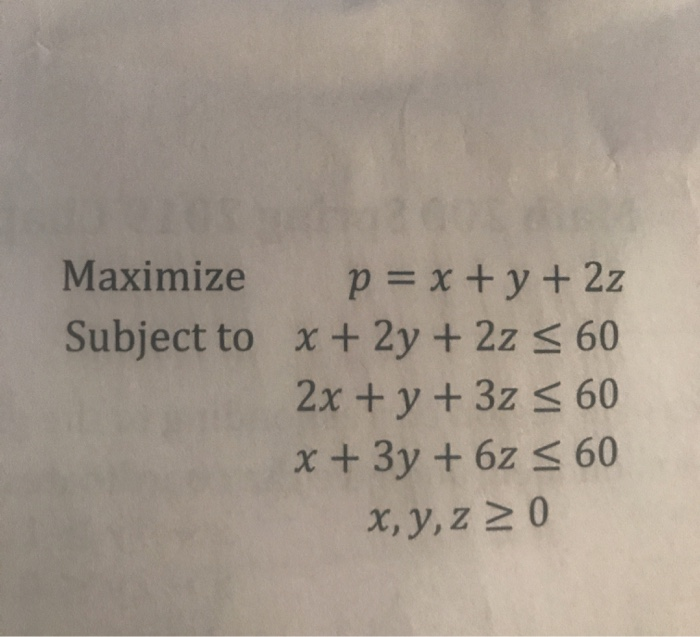p x + y+ 2z Subject to x+ 2y + 2z 60 2x +y + 3z 60 +3y+ 6z s 60 Maximize x, y,z 2 0 p x + y+ 2z Subject to x+ 2y + 2z 60 2x +y + 3z 60 +3y+ 6z s 60 Maximize x, y,z 2 0

• ### 2x-y+2z=8 x+2y+4z=3 x+3y-2z=-1

2x-y+2z=8 x+2y+4z=3 x+3y-2z=-1

• ### solve the system of equation for all variables using a matix x-y-z=1 2x-3y+z=10 x+y-2z=0

solve the system of equation for all variables using a matixx-y-z=12x-3y+z=10x+y-2z=0

• ### 3. Write the following systems of linear equations using augmented matrix form a. 6x+7y= -9 X-y=...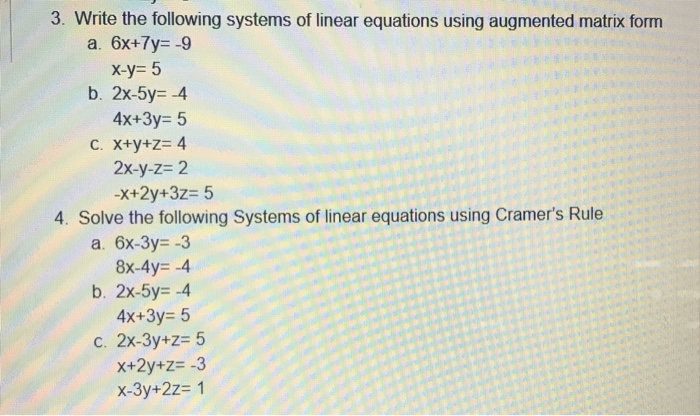3. Write the following systems of linear equations using augmented matrix form a. 6x+7y= -9 X-y= 5 b. 2x-5y= 4 4x+3y= 5 C. x+y+z= 4 2x-y-z= 2 -x+2y+3z= 5 4. Solve the following Systems of linear equations using Cramer's Rule a. 6x-3y=-3 8x-4y= -4 b. 2x-5y= -4 4x+3y= 5 c. 2x-3y+z= 5 X+2y+z= -3 x-3y+2z= 1

• ### solve the system of equation for all variables using a matrix 2x+y+z=6 -3x-4y+2z=4 x=y-z=-2

solve the system of equation for all variables using a matrix2x+y+z=6-3x-4y+2z=4x=y-z=-2

Free Homework App